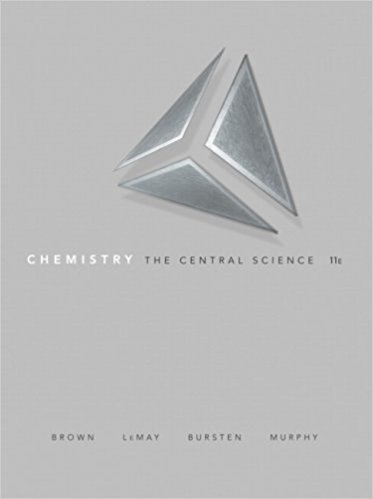×

×

# Solutions for Chapter 19: CHEMICAL THERMODYNAMICS## Full solutions for Chemistry: The Central Science | 11th Edition

ISBN: 9780136006176Solutions for Chapter 19: CHEMICAL THERMODYNAMICS

Solutions for Chapter 19
4 5 0 285 Reviews
16
4
##### ISBN: 9780136006176

Chapter 19: CHEMICAL THERMODYNAMICS includes 98 full step-by-step solutions. This expansive textbook survival guide covers the following chapters and their solutions. This textbook survival guide was created for the textbook: Chemistry: The Central Science , edition: 11. Chemistry: The Central Science was written by and is associated to the ISBN: 9780136006176. Since 98 problems in chapter 19: CHEMICAL THERMODYNAMICS have been answered, more than 56837 students have viewed full step-by-step solutions from this chapter.

Key Chemistry Terms and definitions covered in this textbook

A statement that equal volumes of gases at the same temperature and pressure contain equal numbers of molecules. (Section 10.3)

• calorimetry.

The measurement of heat changes. (6.5)

• deoxyribonucleic acid (DNA)

A polynucleotide in which the sugar component is deoxyribose. (Section 24.10)

• Dipole-dipole interaction

The attraction between the positive end of one dipole and the negative end of another.

• electron density

The probability of finding an electron at any particular point in an atom; this probability is equal to c2, the square of the wave function. Also called the probability density. (Section 6.5)

• Fishhook arrow

A barbed curved arrow used to show the change in position of a single electron.

• Haloalkane (alkyl halide)

A compound containing a halogen atom covalently bonded to an sp3 -hybridized carbon atom. Given the symbol R!X.

• heat of combustion

The heat given off during a reaction in which an alkane reacts with oxygen to produce CO2 and water.

• mass-to-charge ratio(m/z)

Thedetermining factor by which ions are separatedfrom each other in mass spectrometry.

• molal boiling-point-elevation constant (Kb)

A constant characteristic of a particular solvent that gives the increase in boiling point as a function of solution molality: ?Tb = Kbm. (Section 13.5)

• molecular solids

Solids that are composed of molecules. (Sections 12.1 and 12.6)

• Node

A point in space where the value of a wave function is zero

• plastic

A material that can be formed into particular shapes by application of heat and pressure. (Section 12.8)

• protic solvent

A solvent that contains at least one hydrogen atom connected directly to an electronegative atom.

• proton

A positively charged subatomic particle found in the nucleus of an atom. (Section 2.3)

• reaction mechanism

A detailed picture, or model, of how the reaction occurs; that is, the order in which bonds are broken and formed and the changes in relative positions of the atoms as the reaction proceeds. (Section 14.6)

• spectrochemical series

A list of ligands arranged in order of their abilities to split the d-orbital energies (using the terminology of the crystal-field model). (Section 23.6)

• stretching

In IR spectroscopy, atype of vibration that generally produces a signal in the diagnostic region of an IR spectrum.

• symmetrical ether

An ether (R!O!R) where both R groups are identical.

• valence bond theory

A theory that treats a bond as the sharing of electrons that are associated with individual atoms, rather than being associated with the entire molecule.